Next:Unified Multivariate EntropiesUp:Dimensional Divergence Measures and

Properties of M-Dimensional Unified (r,s)-Divergence Measures

In this subsection we shall give some properties of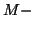dimensional unified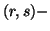measures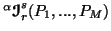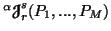and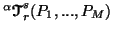(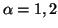and 3).

Property 5.1. The measures(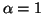and 2),(and 3) and(and 3) are nonnegative for all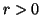and any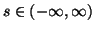are zero iff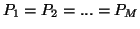. The nonnegativity of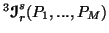follows under the conditions when either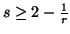or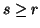with.

Property 5.2. For all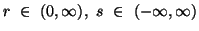, we have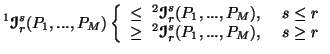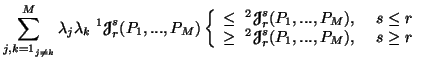and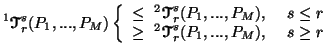Property 5.3. For all, we have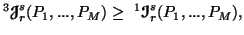and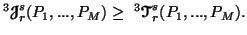Property 5.4. For alland 2, we have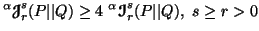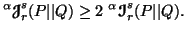Property 5.5. The measures(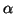=1 and 2),(=1,2 and 3) and(=1,2 and 3) are increasing functions of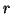(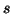fixed) and of(fixed). In particular, when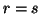, the result still holds.

Property 5.6. For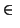(0,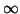),(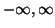),=1 and 2, the measures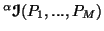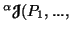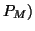and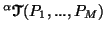are Schur-convex functions of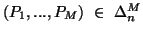i.e.,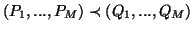implies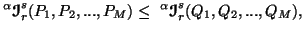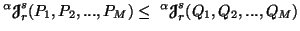and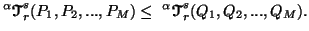Property 5.7. (Generalized data processing inequalities). For(0,),(),=1 and 2, we have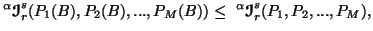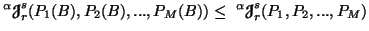and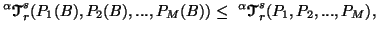where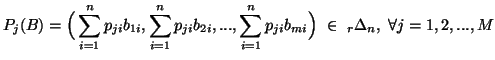with B=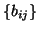,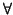i=1,2,...,n; j=1,2,...,m being a stochastic matrix such that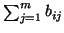=1,i=1,2,...,n.
21-06-2001
Inder Jeet Taneja
Departamento de Matemática - UFSC
88.040-900 Florianópolis, SC - Brazil